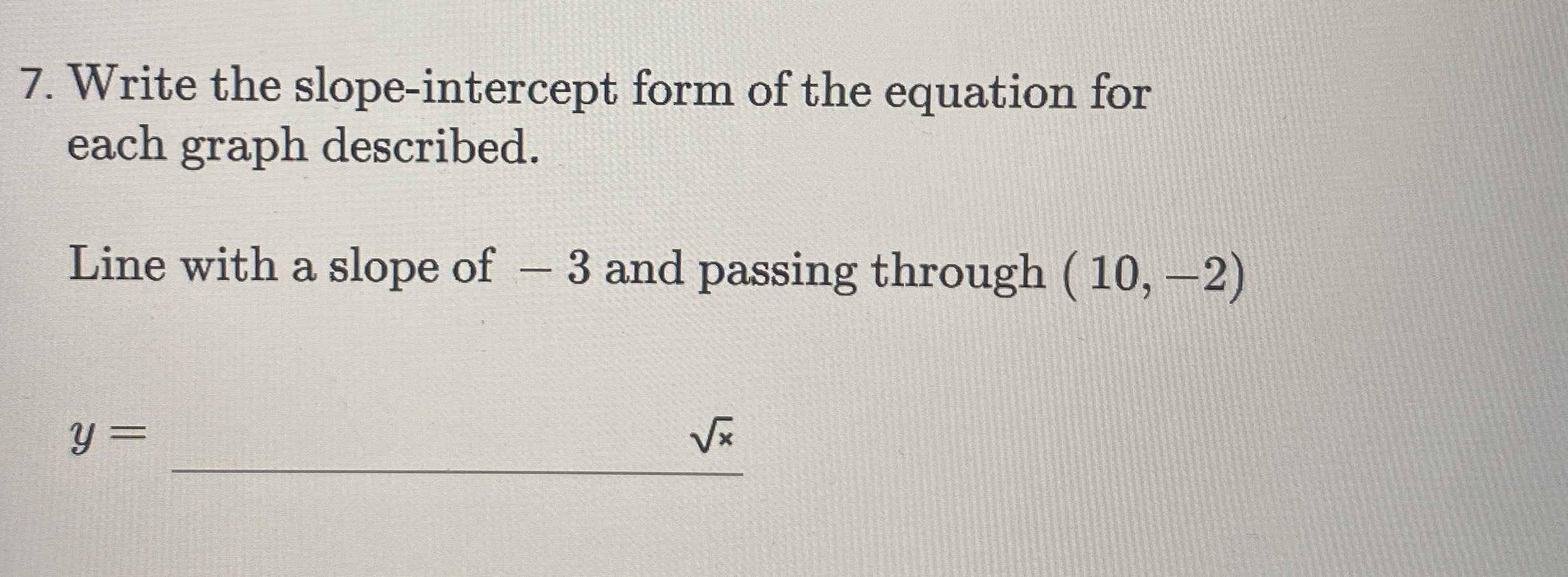### ¿Todavía tienes preguntas de matemáticas?

Pregunte a nuestros tutores expertos
Algebra
Pregunta7. Write the slope-intercept form of the equation for each graph described.

Line with a slope of $$- 3$$ and passing through $$( 10 , - 2 )$$

$$y =$$